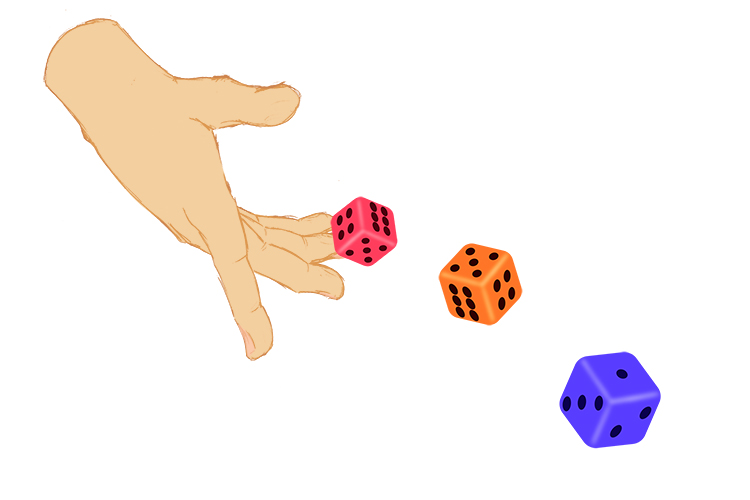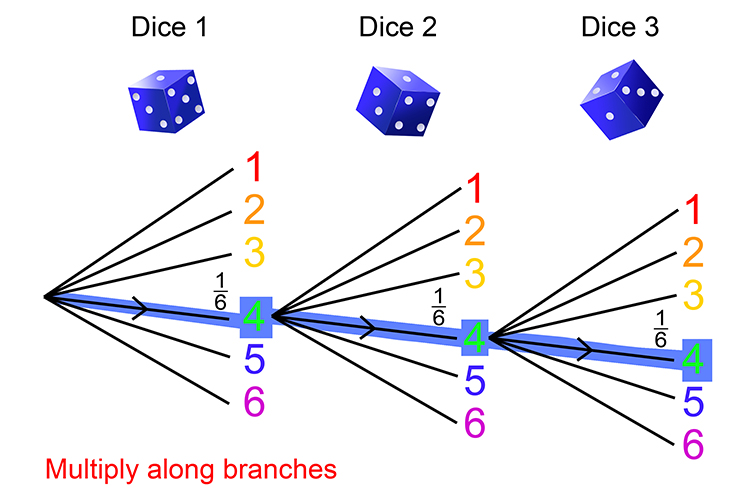# Probability and rolling 3 dice

Always treat the rolling of three dice as 3 separate rolls.

Because in reality that is what happens:The dice will never land at the same time.

If you took an action camera and slowed down the landing of the three dice you would see they would each finish at different times.

Always treat the roll of three dice as three separate rolls. Because in reality that is what happens.

Example

If one throws three dice together on to the ground what is the probability of getting three 4s?

Use

Probability\ =(The\  \n\umber\ of\ ways\ of \ ac\hiev\i\ng\ suc\ess)/(T\he\ \t\otal\ n\umber\ of \ possibl\e\ outcomes

And

Always draw a probability tree.

Roll\i\ng\ a\ 4\ on\ a\ dice,\ probability=1/6\ \overset{(Right)}{\underset{(All\ possibilites)}{text}} ]Probability three number 4s = 1/6\ times\1/6\ times1/6=1/(6\ times6\ times6)=1/(216)

Probability = 1 in 216 chance  or = 0.004630

And if 1 = 100%             then 0.004630=0.46% chance